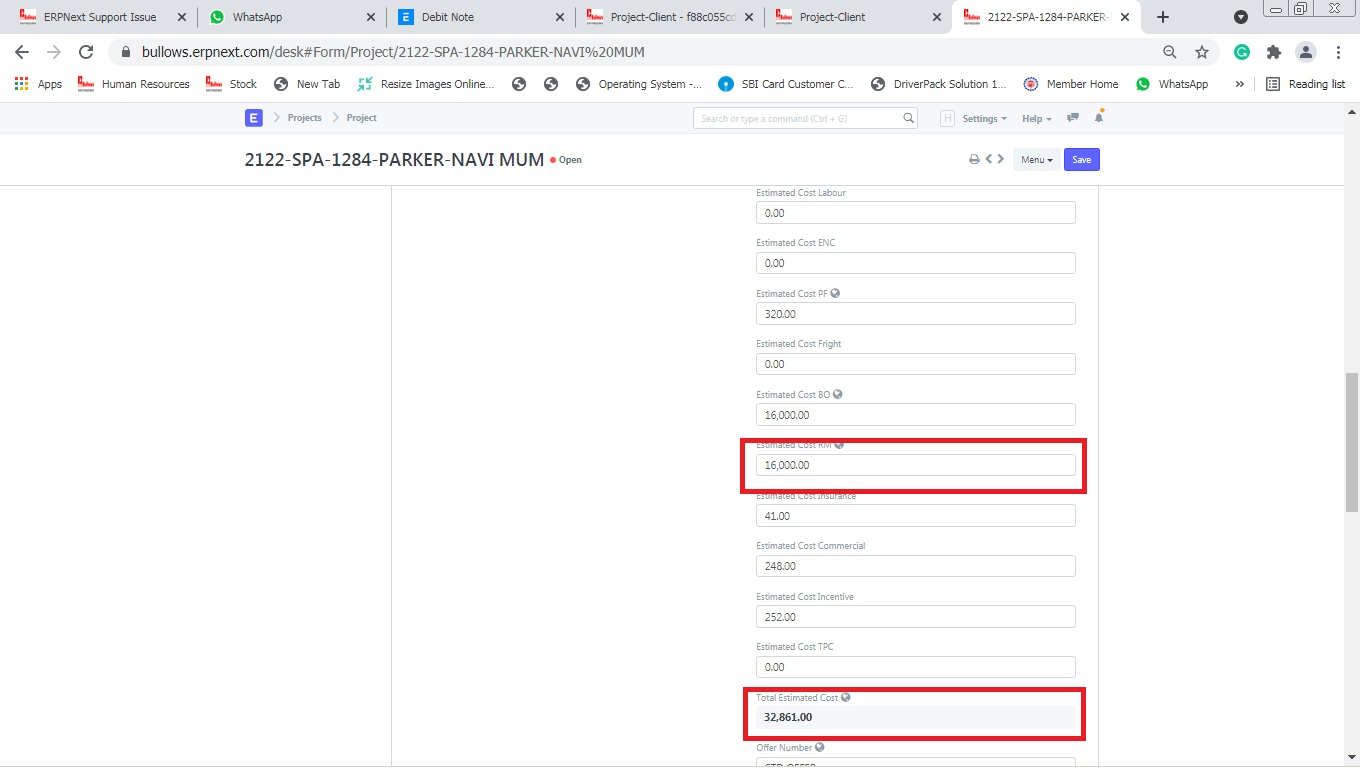# Project Doctype Basic Calculation Error

Hello

I found the issue in Project Doctype Please Check Below Example

I have created One Project, Project id is 2122-SPA-1284-PARKER-NAVI MUM when I entered 1Rs in Estimated Cost Rm, Then Total Estimation Cost, Estimation Gross Margin,
Estimation Gross Margin, Percentage Values are Correct.

If I have Entered 0 Rs In Estimated Cost Rm Then the Value is Not showing Correct Please refer below screenshot

After Saving Above Doc . the value in Estimated Cost RM Its shows repeated from above Fieldand Total Estimation Cost, Estimation Gross Margin, Estimation Gross Margin, Percentage Values are Not showing Correct.I have Created Custom Script for the Above Calculation.

Earlier Below Custom Script working Properly.

frappe.ui.form.on(‘Project’, {

validate: function(frm) {

if (!frm.doc.est_material_cost && frm.doc.est_material_cost !== 0) {

frm.set_value(‘est_material_cost’, frm.doc.estimated_cost_bo);

}

let total_estimated_cost = frm.doc.est_material_cost + frm.doc.estimated_cost_bo + frm.doc.estimated_cost_labour + frm.doc.estimated_cost_enc + frm.doc.estimated_cost_pf + frm.doc.estimated_cost_fright + frm.doc.estimated_cost_insurance + frm.doc.estimated_cost_commercial + frm.doc.estimated_cost_incentive;

let estimated_gross_margin = frm.doc.estimated_costing - total_estimated_cost;

let estimated_gross_margin_percentage = (estimated_gross_margin / frm.doc.estimated_costing) * 100;

frm.set_value(‘estimated_gross_margin’, estimated_gross_margin);

frm.set_value(‘estimated_gross_margin_percentage’, estimated_gross_margin_percentage);

frm.set_value(‘total_estimated_cost’, total_estimated_cost);

}

})